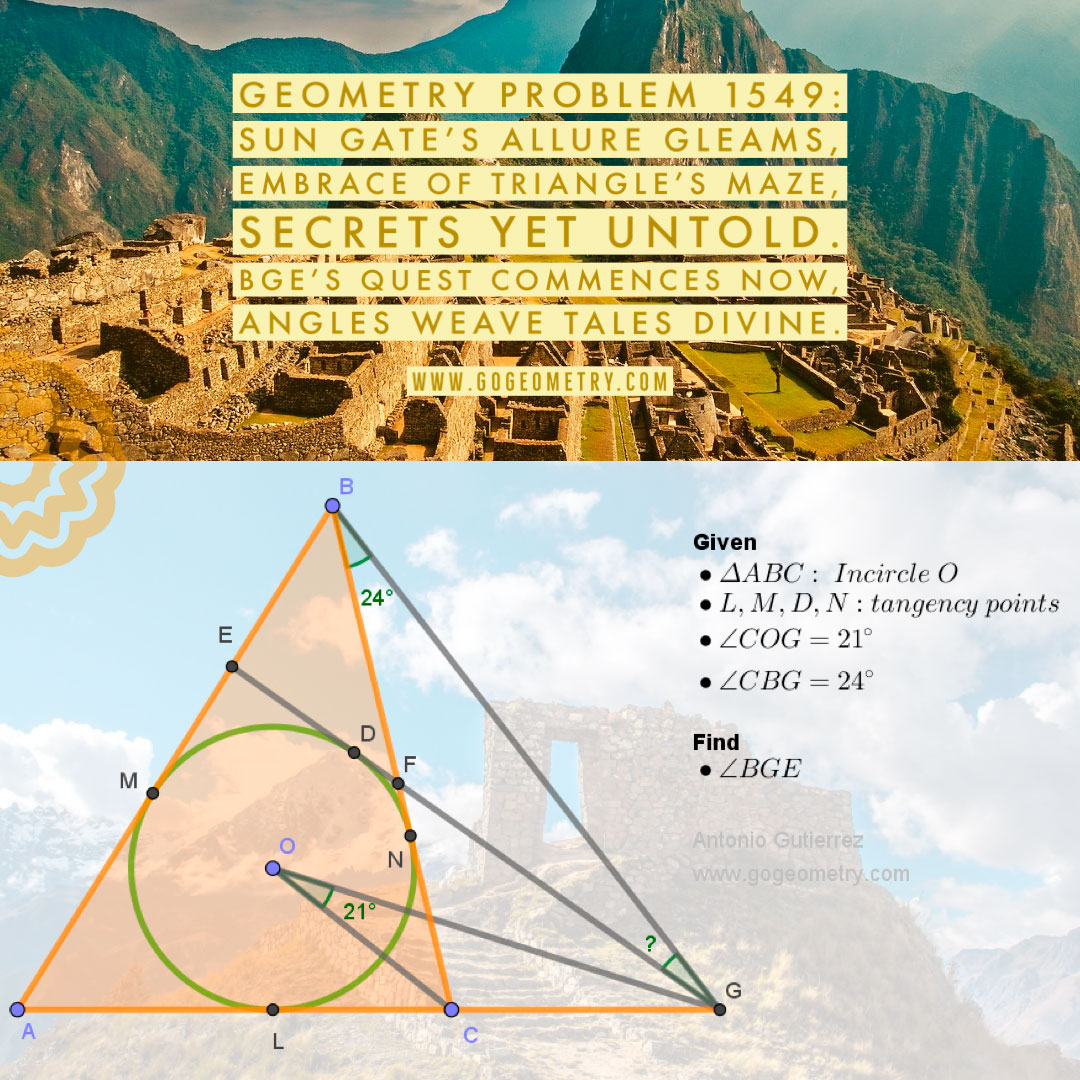# Geometry Problem 1549: Unraveling the Geometric Mystery: Calculating Angle BGE with the Incircle and Tangent in Triangle ABC: A High School Level Investigation

In triangle ABC, a circle with center O is inscribed and is tangent to sides AC, AB, and BC at points L, M, and N, respectively. Let D be a point on the arc MN, and from D, draw the tangent that intersects AB at point E, BC at point F, and the extension of AC at point G. Given that the angle COG measures 21 degrees and the angle CBG measures 24 degrees, calculate the measure of angle BGE.Amidst points and arcs,
Inscribed circle's dance begins,
Angles weave their tale.

## Lines and Shadows: Exploring Problem 1549 with the Sun Gate (Inti Punku) at Machu Picchu as the Backdrop